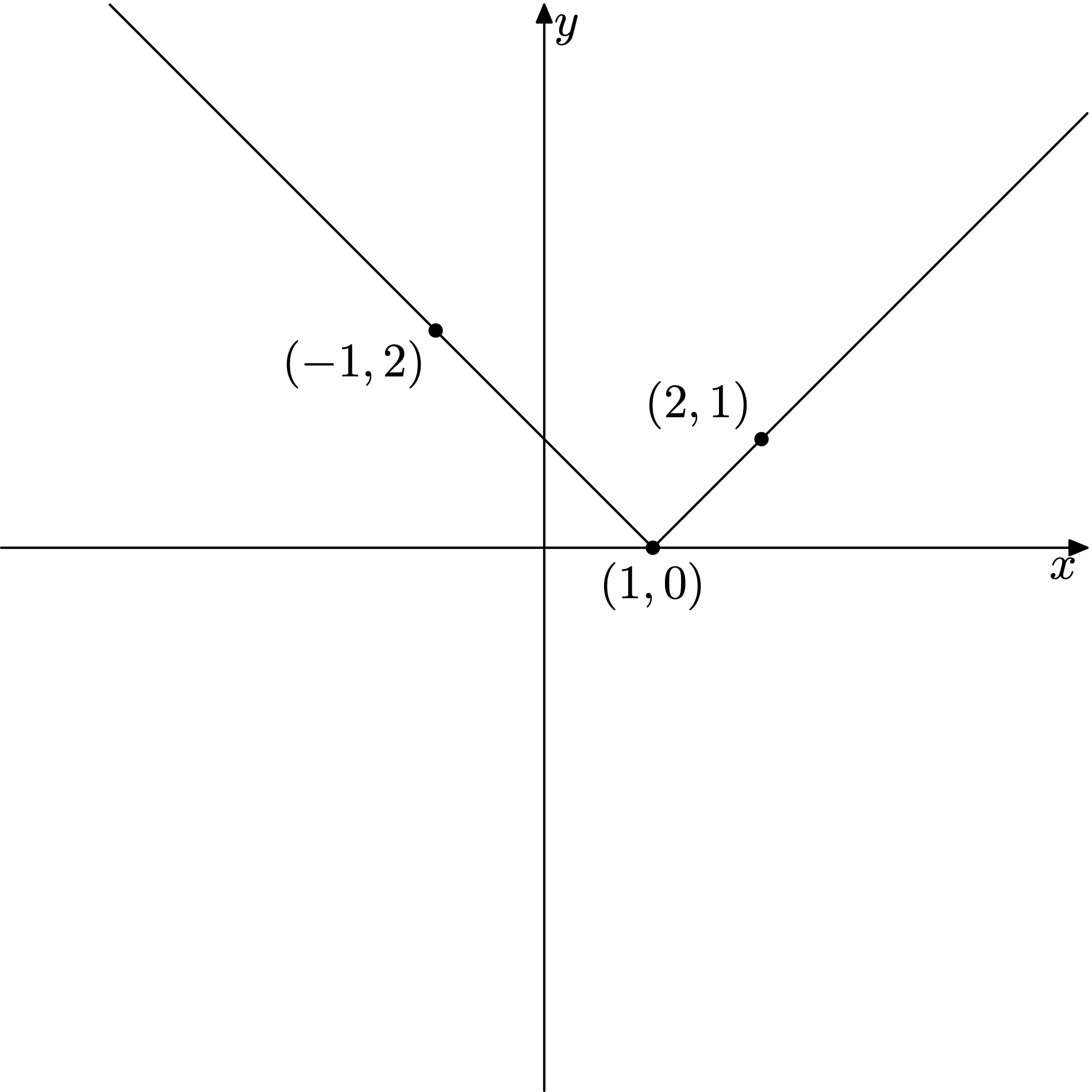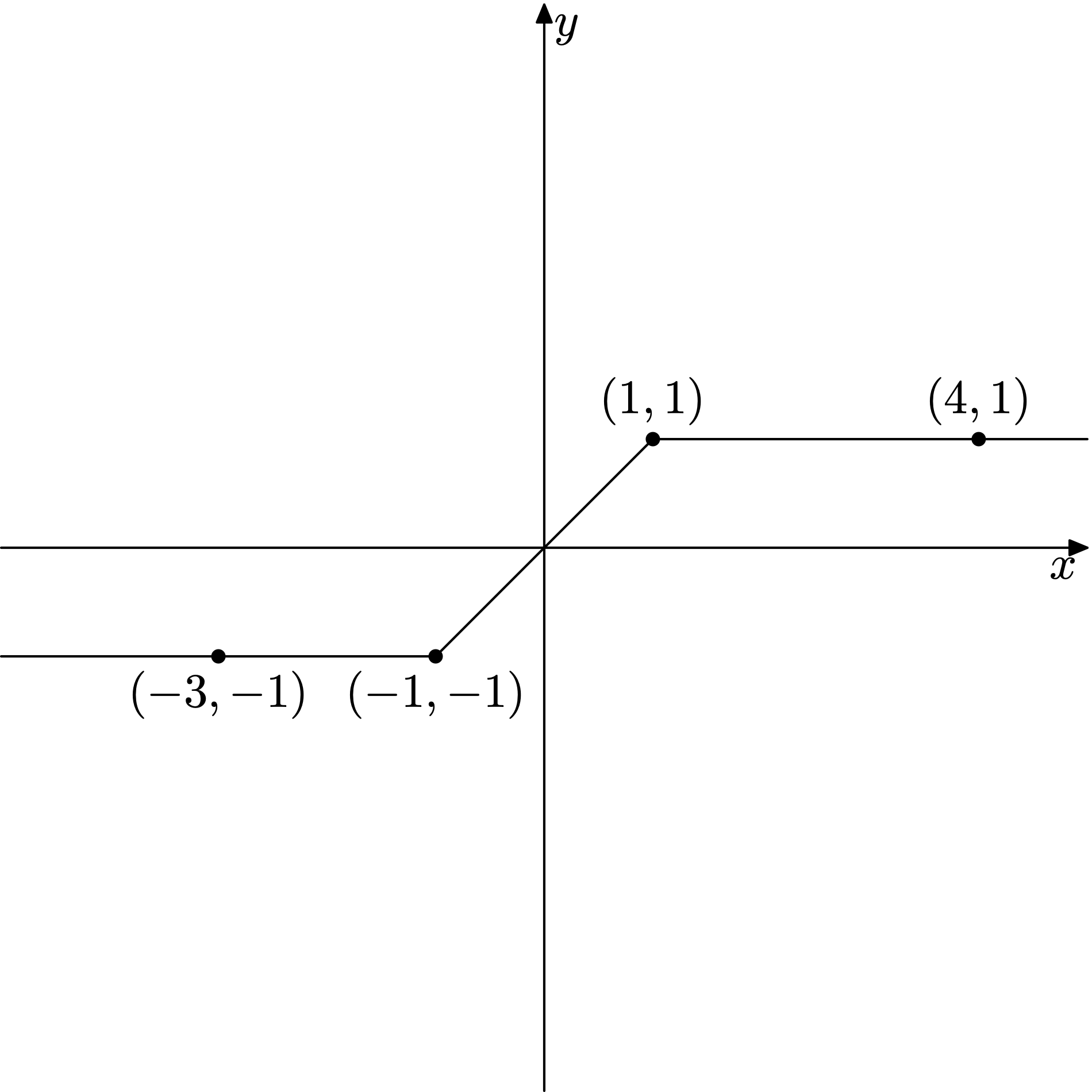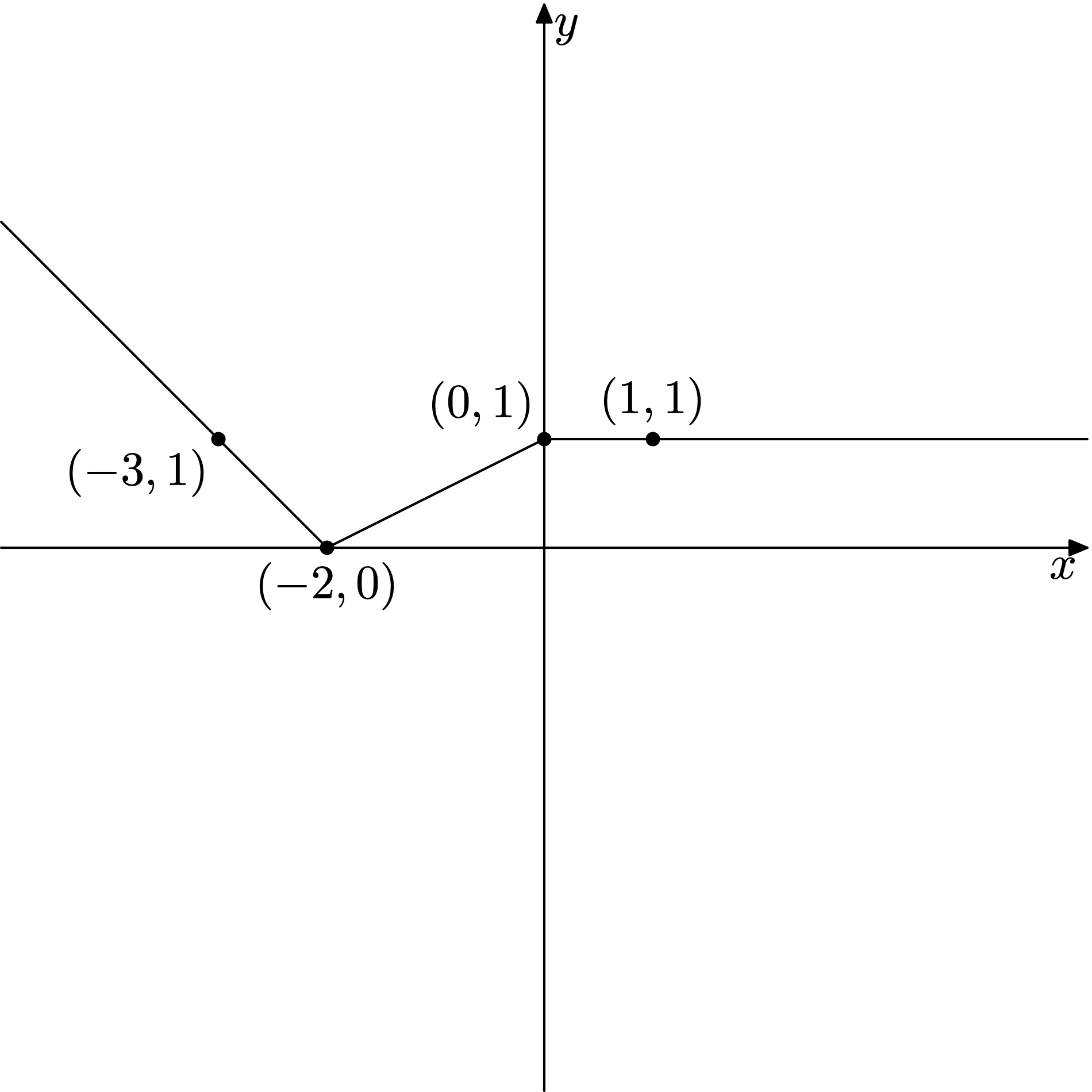시간 제한 메모리 제한 제출 정답 맞은 사람 정답 비율
1 초 512 MB 1 1 1 100.000%

## 문제

Alice is obsessed with linear functions and especially their plots that are always so mysteriously straight. Recently she found out a plot of function $f(x) = |x-1|$ that impressed her a lot: it was twice as mysterious and beautiful since it consisted not only of one straight-line segment, but of two of them!

Alice immediately thought of a function that is $n \geq 2$ times as mysterious as a linear function. Formally, she came up with a piecewise linear function $f(x)$, whose plot consists of $n$ straight-line segments. Function $f(x)$ is defined by $n+1$ points $P_0, P_1, \ldots, P_{n-1}, P_n$ belonging to its plot and allowing to reconstruct it in a following manner. Plot of function $f(x)$ is a polyline consisting of two rays $P_1 P_0$, $P_{n-1} P_n$ and $n-2$ line segments $P_1 P_2$, $\ldots$, $P_{n-2} P_{n-1}$. Each point $P_i$ is defined by its Cartesian coordinates $(x_i, y_i)$, which are both integers. It is guaranteed that $x_i > x_{i-1}$ for all $i$ between $1$ and $n$, i.e. given polyline is a plot of some function $f(x)$. Please, refer to the Note section for more details.

Now Alice asks you if it is possible to express her function $f(x)$ as a linear combination of terms of form $|x - a_i|$. Formally, your task is to find out if there exist two finite sequences of real numbers $\lambda_1, \lambda_2, \ldots, \lambda_m$ and $a_1, a_2, \ldots, a_m$ such that the following equation holds:

$$f(x) = \sum\limits_{i=1}^m \lambda_i |x - a_i|$$

## 입력

First line of input contains an integer $n$ ($2 \leq n \leq 100\,000$), the number of segments in a polyline that is a plot of Alice function.

In the $i$-th of next $n+1$ lines (indexed from zero) there are two integers $x_i$, $y_i$ ($-10^6 \leq x_i, y_i \leq 10^6$), coordinates of point $P_i$.

It is guaranteed that $x_0 < x_1 < \ldots < x_n$.

## 출력

If it is possible to express $f(x)$ as a linear combination of terms of form $|x - a_i|$, print the only word "Yes" (without quotes). Otherwise print the only word "No" (without quotes).

## 예제 입력 1

2
-1 2
1 0
2 1


## 예제 출력 1

Yes


## 예제 입력 2

3
-3 -1
-1 -1
1 1
4 1


## 예제 출력 2

Yes


## 예제 입력 3

3
-3 1
-2 0
0 1
1 1


## 예제 출력 3

No


## 힌트

Pictures for the sample cases are given below: